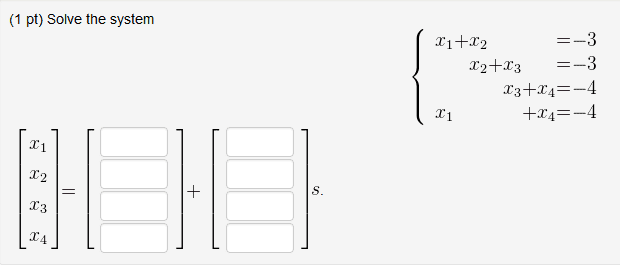# Linear Algebra: Solving a system with free variables

Here is a picture of the problem.Can anyone give me some hints on the problem? I've looked in my textbook, but I don't know what "s" means. I found stuff on the parametric vector form, and it gives me the equation x = su + tv, but I don't see any "t"'s in this problem.

I first tried setting up an augmented matrix with

[ 1 1 0 0 | -3 ]
[ 0 1 1 0 | -3 ]
[ 0 0 1 1 | -4 ]
[ 1 0 0 1 | -4 ]

And I solved for the four variables, like:
x1 = -3 - x2
x2 = -3 - x3
x3 = -4 - x4
x4 = -4 - x1

And now I'm stumped. I didn't think these would do anything, but setting the first and second rows equal to each other, and the third and fourth rows equal to each other simply gets x1 - x3 = 0 for both of them.

I didn't think this would do anything either, but I tried also back substituting the x1 equation into the x4 equation, and then the x2 equation into x4, and so on, and that ended up with x4 = x4.

edit: never my idea didn't work

edit: never my idea didn't work

Thanks for the try though.

Mark44
Mentor
Here is a picture of the problem.Can anyone give me some hints on the problem? I've looked in my textbook, but I don't know what "s" means. I found stuff on the parametric vector form, and it gives me the equation x = su + tv, but I don't see any "t"'s in this problem.

I first tried setting up an augmented matrix with

[ 1 1 0 0 | -3 ]
[ 0 1 1 0 | -3 ]
[ 0 0 1 1 | -4 ]
[ 1 0 0 1 | -4 ]

And I solved for the four variables, like:
x1 = -3 - x2
x2 = -3 - x3
x3 = -4 - x4
x4 = -4 - x1
It looks like you didn't actually do anything with your augmented matrix. Use row reduction to get the matrix in reduced row echelon form. When it's completely reduced you can solve for x1, x2, and x3 in terms of x4. Your solution will look like a vector + a parameter (that's s) times another vector.
Ryry013 said:
And now I'm stumped. I didn't think these would do anything, but setting the first and second rows equal to each other, and the third and fourth rows equal to each other simply gets x1 - x3 = 0 for both of them.

I didn't think this would do anything either, but I tried also back substituting the x1 equation into the x4 equation, and then the x2 equation into x4, and so on, and that ended up with x4 = x4.

HallsofIvy
$$\begin{bmatrix} 1 & 0 & 0 & a_1 & b_1 \\ 0 & 1 & 0 & a_2 & b_2 \\ 0 & 0 & 1 & a_3 & b_3 \\ 0 & 0 & 0 & 0 & 0\end{bmatrix}$$
That is equivalent to the equation $x_1+ a_1x_4= b_1$, $x_2+ a_2x_4= b_4$, and $x_3+ a_3x_4= b_4$ which can be solved as "$a_i$ equals a number plus a multiple of $x_4$".